SBVS233B January   2016  – June 2021

PRODUCTION DATA

1. Features
2. Applications
3. Description
4. Revision History
5. Pin Configurations and Functions
6. Specifications
7. Detailed Description
1. 7.1 Overview
2. 7.2 Functional Block Diagram
3. 7.3 Feature Description
4. 7.4 Device Functional Modes
8. Application and Implementation
1. 8.1 Application Information
2. 8.2 Typical Applications
1. 8.2.1 Low-Input, Low-Output (LILO) Voltage Conditions
2. 8.2.2 Typical Application for a 5.0-V Rail
9. Power Supply Recommendations
10. 10Layout
1. 10.1 Layout Guidelines
2. 10.2 Layout Example
11. 11Device and Documentation Support
1. 11.1 Device Support
1. 11.1.1 Development Support
2. 11.1.2 Device Nomenclature
2. 11.2 Documentation Support
4. 11.4 Support Resources
6. 11.6 Electrostatic Discharge Caution
7. 11.7 Glossary
12. 12Mechanical, Packaging, and Orderable Information

• RGR|20
• RGR|20

## 6.6 Typical Characteristics

at TA = 25°C, VIN = 1.4 V or VIN = VOUT(NOM) + 0.4 V (whichever is greater), VBIAS = open, VOUT(NOM) = 0.8 V, VEN = 1.1 V, COUT = 47 μF, CNR/SS = 0 nF, CFF = 0 nF, and PG pin pulled up to VIN with 100 kΩ (unless otherwise noted)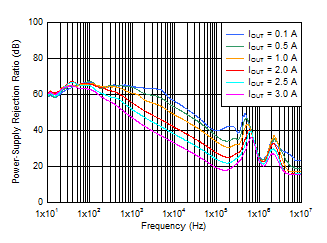VIN = 1.1 V, VBIAS = 5 V, COUT = 47 μF || 10 μF || 10 μF, CNR/SS = 10 nF, CFF = 10 nF
Figure 6-1 PSRR vs Frequency and IOUT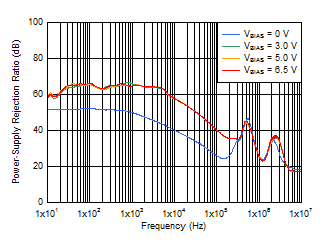VIN = 1.4 V, IOUT = 1 A, COUT = 47 μF || 10 μF || 10 μF, CNR/SS = 10 nF, CFF = 10 nF
Figure 6-3 PSRR vs Frequency and VBIAS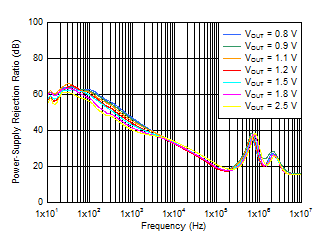VIN = VOUT + 0.3 V, VBIAS = 5.0 V, IOUT = 3 A, COUT = 47 μF || 10 μF || 10 μF, CNR/SS = 10 nF, CFF = 10 nF
Figure 6-5 PSRR vs Frequency and VOUT With Bias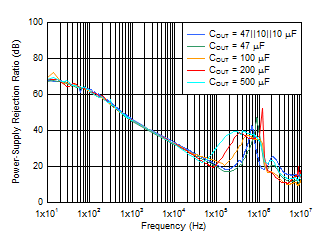VIN = VOUT + 0.3 V, VOUT = 1 V, IOUT = 3 A, CNR/SS = 10 nF, CFF = 10 nF
Figure 6-7 PSRR vs Frequency and COUT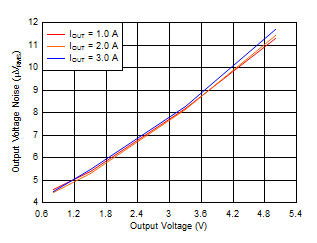VIN = VOUT + 0.3 V and VBIAS = 5 V for VOUT ≤ 2.2 V, COUT = 47 μF || 10 μF || 10 μF, CNR/SS = 10 nF, CFF = 10 nF, RMS noise BW = 10 Hz to 100 kHz
Figure 6-9 Output Voltage Noise vs Output Voltage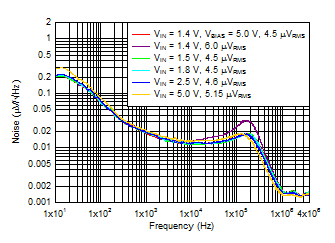IOUT = 3 A, COUT = 47 μF || 10 μF || 10 μF, CNR/SS = 10 nF, CFF = 10 nF, RMS noise BW = 10 Hz to 100 kHz
Figure 6-11 Output Noise vs Frequency and Input VoltageVIN = VOUT + 0.3 V, VBIAS = 5 V, IOUT = 3 A, sequencing with a dc/dc converter and PG, COUT = 47 μF || 10 μF || 10 μF, CNR/SS = 10 nF, RMS noise BW = 10 Hz to 100 kHz
Figure 6-13 Output Noise vs Frequency and CFF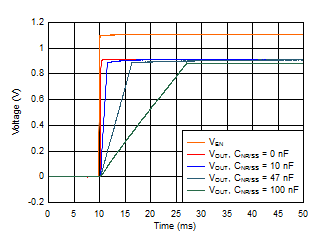VIN = 1.2 V, VOUT = 0.9 V, VBIAS = 5.0 V, IOUT = 3 A, COUT = 47 μF || 10 μF || 10 μF, CFF = 10 nF
Figure 6-15 Start-Up Waveform vs Time and CNR/SS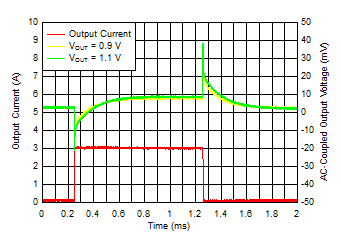IOUT, DC = 100 mA, COUT = 47 μF || 10 μF || 10 μF, CNR/SS = CFF = 10 nF, slew rate = 1 A/μs
Figure 6-17 Load Transient vs Time and VOUT Without Bias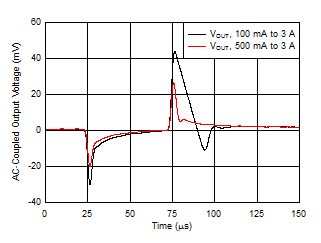VIN = 1.2 V, VBIAS = 5.0 V, COUT = 47 μF || 10 μF || 10 μF, CNR/SS = CFF = 10 nF, slew rate = 1 A/μs
Figure 6-19 Load Transient vs Time and DC Load (VOUT = 0.9 V)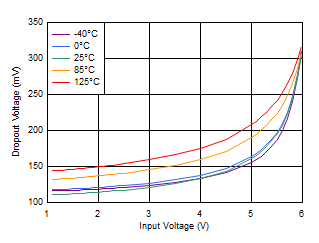IOUT = 3 A, VBIAS = 6.5 V
Figure 6-21 Dropout Voltage vs Input Voltage With BiasVIN = 1.1 V, VBIAS = 3 V
Figure 6-23 Dropout Voltage vs Output Current With Bias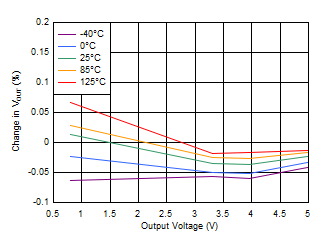IOUT = 100 mA to 3 A
Figure 6-25 Load Regulation vs Output VoltageVIN = 3.8 V
Figure 6-27 Load Regulation (3.3-V Output)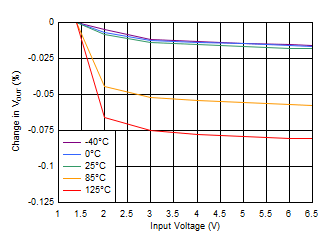VOUT = 0.8 V, VBIAS = 0 V, IOUT = 5 mA
Figure 6-29 Line Regulation Without Bias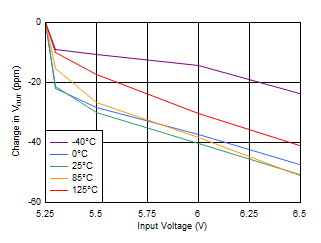IOUT = 5 mA
Figure 6-31 Line Regulation (5-V Output)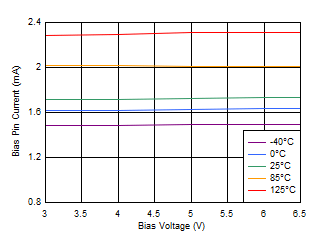VIN = 1.1 V, IOUT = 5 mA
Figure 6-33 Quiescent Current vs Bias Voltage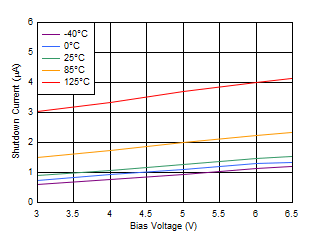VIN = 1.1 V
Figure 6-35 Shutdown Current vs Bias Voltage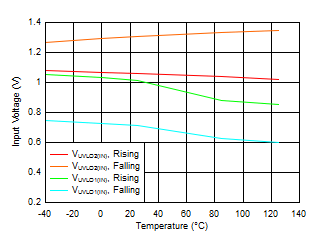Figure 6-37 VIN UVLO vs Temperature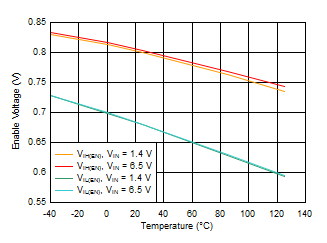VIN = 1.4 V, 6.5 V
Figure 6-39 Enable Threshold vs Temperature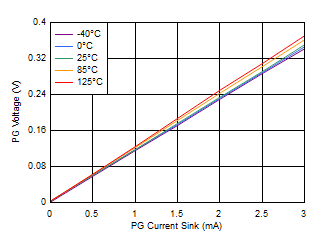VIN = 6.5 V
Figure 6-41 PG Voltage vs PG Current Sink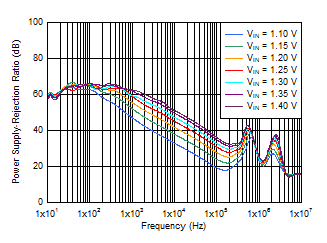IOUT = 3 A, VBIAS = 5 V, COUT = 47 μF || 10 μF || 10 μF, CNR/SS = 10 nF, CFF = 10 nF
Figure 6-2 PSRR vs Frequency and VIN With Bias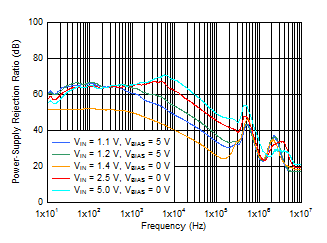IOUT = 1 A, COUT = 47 μF || 10 μF || 10 μF, CNR/SS = 10 nF, CFF = 10 nF
Figure 6-4 PSRR vs Frequency and VIN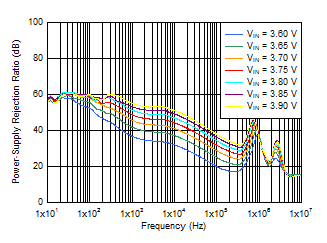IOUT = 3 A, COUT = 47 μF || 10 μF || 10 μF, CNR/SS = 10 nF, CFF = 10 nF
Figure 6-6 PSRR vs Frequency and VIN for VOUT = 3.3 V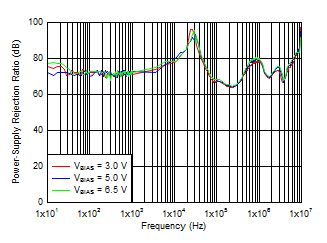VIN = VOUT + 0.3 V, VOUT = 1 V, IOUT = 3 A, COUT = 47 μF || 10 μF || 10 μF, CNR/SS = 10 nF, CFF = 10 nF
Figure 6-8 VBIAS PSRR vs Frequency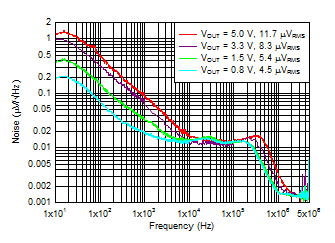VIN = VOUT + 0.3 V and VBIAS = 5 V for VOUT ≤ 2.2 V, IOUT = 3 A, COUT = 47 μF || 10 μF || 10 μF, CNR/SS = 10 nF, CFF = 10 nF, RMS noise BW = 10 Hz to 100 kHz
Figure 6-10 Output Noise vs Frequency and Output Voltage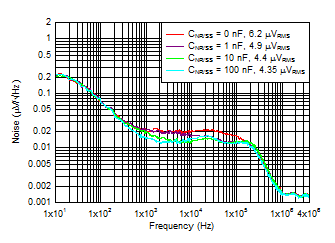VIN = VOUT + 0.3 V, VBIAS = 5 V, IOUT = 3 A, COUT = 47 μF || 10 μF || 10 μF, CFF = 10 nF, RMS noise BW = 10 Hz to 100 kHz
Figure 6-12 Output Noise vs Frequency and CNR/SS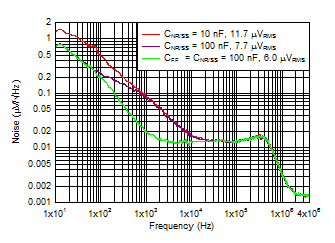IOUT = 3 A, COUT = 47 μF || 10 μF || 10 μF, CFF = 10 nF, RMS noise BW = 10 Hz to 100 kHz
Figure 6-14 Output Noise at 5.0-V Output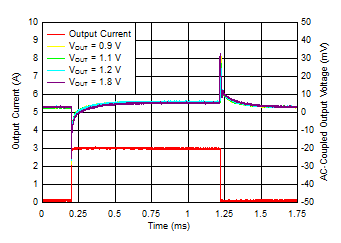VIN = VOUT + 0.3 V, VBIAS = 5 V, IOUT, DC = 100 mA, slew rate = 1 A/μs, CNR/SS = CFF = 10 nF, COUT = 47 μF || 10 μF || 10 μF
Figure 6-16 Load Transient vs Time and VOUT With Bias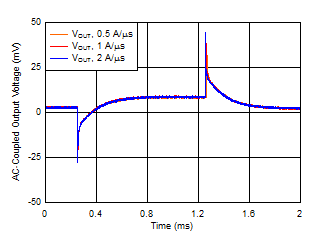VOUT = 5 V, IOUT, DC = 100 mA, IOUT = 100 mA to 3 A, COUT = 47 μF || 10 μF || 10 μF, CNR/SS = CFF = 10 nF
Figure 6-18 Load Transient vs Time and Slew Rate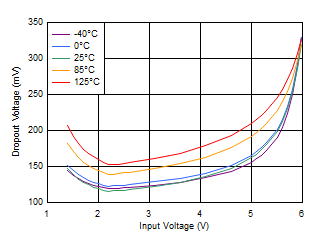IOUT = 3 A, VBIAS = 0 V
Figure 6-20 Dropout Voltage vs Input Voltage Without Bias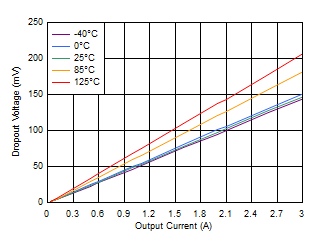VIN = 1.4 V, VBIAS = 0 V
Figure 6-22 Dropout Voltage vs Output Current Without Bias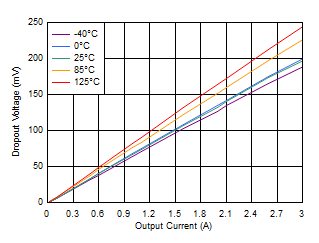VIN = 5.5 V
Figure 6-24 Dropout Voltage vs Output Current (High VIN)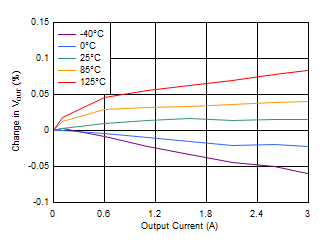VIN = 1.4 V, VBIAS = 0 V
Figure 6-26 Load Regulation With Bias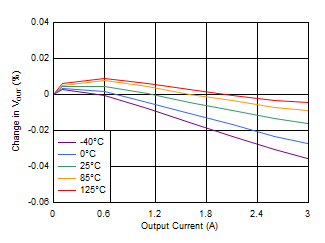VIN = 5.5 V
Figure 6-28 Load Regulation (5-V Output)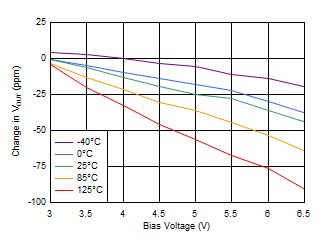VOUT = 0.8 V, VIN = 1.1 V, IOUT = 5 mA
Figure 6-30 Line Regulation Without Bias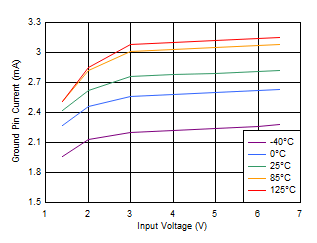VBIAS = 0 V, IOUT = 5 mA
Figure 6-32 Quiescent Current vs Input Voltage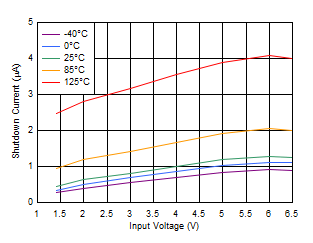VBIAS = 0 V
Figure 6-34 Shutdown Current vs Input Voltage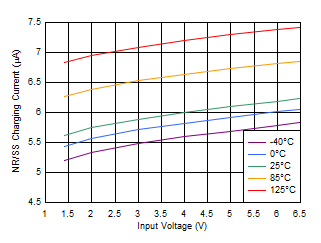VBIAS = 0 V
Figure 6-36 INR/SS Current vs Input VoltageVIN = 1.1 V
Figure 6-38 VBIAS UVLO vs Temperature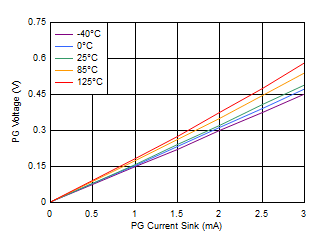Figure 6-40 PG Voltage vs PG Current Sink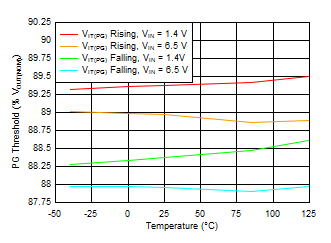Figure 6-42 PG Threshold vs Temperature